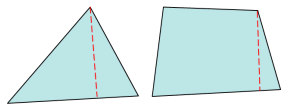# Abaqus

Abaqus-specific checks used to calculate element quality for 2D and 3D elements.

## 2D and 3D Element Checks

These checks apply to both types of elements, but when applied to 3D elements they are generally applied to each face of the element. The value of the worst face is reported as the 3D element’s overall quality value.

Additional element checks not listed here are not part of the solver’s normal set of checks, and therefore use HyperMesh check methods.
Aspect Ratio
Ratio of the longest edge of an element to its shortest edge.
When applied to 3D elements, the same method is used (longest edge divided by shortest edge) rather than evaluating each face individually and taking the worst face result.
Interior Angles
Maximum and minimum values are evaluated independently for triangles and quadrilaterals.
Jacobian
Deviation of an element from its ideal or "perfect" shape, such as a triangle’s deviation from equilateral. The Jacobian value ranges from 0.0 to 1.0, where 1.0 represents a perfectly shaped element. The determinant of the Jacobian relates the local stretching of the parametric space which is required to fit it onto the global coordinate space.
HyperMesh evaluates the determinant of the Jacobian matrix at each of the element’s integration points, also called Gauss points, or at the element’s corner nodes, and reports the ratio between the smallest and the largest. In the case of Jacobian evaluation at the Gauss points, values of 0.7 and above are generally acceptable. You can select which method of evaluation to use (Gauss point or corner node) from the Check Element settings.
Length (min)
Minimum element lengths are calculated using one of two methods:
• The shortest edge of the element. This method is used for non-tetrahedral 3D elements.
• The shortest distance from a corner node to its opposing edge (or face, in the case of tetra elements); referred to as "minimal normalized height".Skew (tria only)
Defined by shape factor. Abaqus determines triangular element shape factor by dividing the element’s area by the area of an ideally shaped element. The ideally shaped element is defined as an equilateral triangle with the same circumradius—the radius of a circle that passes through the three vertices of the triangle—as the element.
This shape factor converts to skew by subtracting it from 1. Thus, a perfect equilateral tria element has a skew of 0 and the worst tria has a value of 1.0.
Quadrilaterals are simply assigned a value of 0.

## 3D Element Only Checks

Volume Skew
Only applicable to tetrahedral elements; all others are assigned values of zero.
Volume Skew is defined as 1 minus the shape factor, so a skew of 0 is perfect and a skew of 1 is the worst possible value.
The shape factor for a tetrahedral element is determined by dividing the element’s volume by the volume of an ideal (equilateral) tetrahedron of the same circumradius. In the case of tetrahedral elements, the circumradius is the radius of a sphere passing through the four vertices of the tetrahedron.